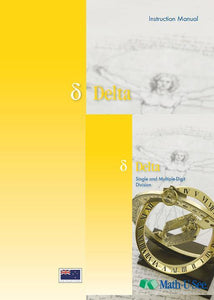or 6 weekly interest-free payments from \$12.50 withwhat's this?Demme Learning

# Math.U.See Delta

Regular price \$65.00 \$40.63 Unit price per
Tax included. Shipping calculated at checkout.

## Math.U.See Delta

#### Division for single and multiple-digit numbers and other topics.

Division is presented as the inverse of multiplication. Single-digit division facts are learned and the concepts of division and place value are applied when solving long division problems.

Major Concepts and Skills Include:

• Using strategies based on place value and properties of operations to divide
• Understanding division as solving for an unknown factor
• Fluently dividing any combination of whole numbers
• Solving abstract and real-world problems involving all four operations
• Interpreting remainders in short and long division
• Understanding fraction notation in light of division

• Reading and writing Roman numerals
• Dividing, multiplying, adding, and subtracting NZ currency and standard units of measure
• Understanding angle measure and geometric shapes including points, segments, rays, and lines
• Classifying shapes based on defining attributes
• Understanding and computing area and volume

Delta Instruction Pack includes: Instruction Manual & DVD

Delta Student pack includes: Student Workbook & Test & Activity booklet.

Grab an extra Student Pack for an additional Student.

For a complete set to get started with Math.U.See you will need to purchase the Integer Block set (a one of purchase for use with ALL levels of Math.U.See), the Delta instruction pack & Delta Student Pack